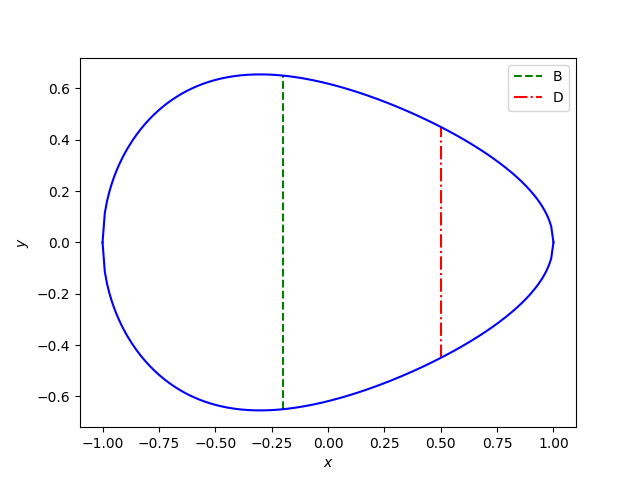# Empirical formula for the shape of an egg

A while back I wrote about a simple equation for the shape of an egg. That equation is useful for producing egg-like images, but it’s not based on extensive research into actual eggs.

I recently ran across a more realistic, but also more complicated, equation for modeling the shape of real eggs . The equation models the shape of an egg by rotating the graph of a function of the form

y = (B/2) f(x; L, w, D)

around the x-axis. The parameters B and L are simple to describe, but w and D take a little more explanation.

## Parameters

Imagine the egg positioned so the x-axis runs along the center of the egg, with the fat end on the left at –L/2 and the pointy end on the right at L/2. So L is the length of the egg.

The parameter B is the maximum breadth of the egg, the maximum diameter perpendicular to the central axis. If you plot y above as a function of x, the maximum value of y is B/2.

The authors describe w as “the parameter that shows the distance between two vertical axes corresponding to the maximum breadth and the half length of the egg.” I find that description confusing. I believe they’re saying that –w is the location of the maximum of y. So B/2 is the maximum value of y, and –w is where that maximum occurs.

The authors define a parameter DL/4 that I’m calling D for simplicity. They define this parameter as the “egg diameter at the point of L/4 from the pointed end.” That is, D is twice the value of y at x = L/4. Recall that the egg runs from –L/2 to L/2, so we’re talking about the point 3/4 of the way between the fat end and the pointed end.

To summarize, L, B, w, and D are the length, maximum breadth, the negative of the location of the maximum breadth, and the breadth three quarters of the way between the fat and pointed ends.

## Equation

With all that out of the way, here’s the equation. I’ll write it up in Python, but I wanted to include a screen shot from the paper in case I made an error in coding it up.## Python implementation

Here’s Python code that implements this function, if I haven’t made any errors.

```    from numpy import sqrt

def egg_radius(x, L, B, w, D):

# Note that k does not involve x
k = sqrt(5.5*L**2 + 11*L*w + 4*w**2)
k *= sqrt(3)*B*L - 2*D*sqrt(L**2 + 2*w*L + 4*w**2)
k /= sqrt(3)*B*L
k /= sqrt(5.5*L**2 + 11*L*w + 4*w*w) - 2*sqrt(L**2 + 2*w*L + 4*w**2)

# coefficients of a quadratic in x
a = 2*(L-2*w)
b = L**2 + 8*L*w - 4*w**2
c = 2*L*w**2 + L**2*w + L**3

t = L*(L**2 + 8*w*x + 4*w**2)
t /= a*x**2 + b*x + c

y = 0.5*B*sqrt(L**2 - 4*x**2)/sqrt(L**2 + 8*w*x + 4*w**2)
y *= (1 - k*(1 - sqrt(t)))

return y
```

Here’s a plot using this code:And here’s the code that was used to make the plot:

```    from numpy import linspace
import matplotlib.pyplot as plt

L = 2
B = 1.3
w = 0.2
D = 0.9

plt.plot([-w, -w], [-B/2, B/2], 'g--')
z = egg_radius(L/4, L, B, w, D)
plt.plot([L/4, L/4], [-z, z], "r-.")
x = linspace(-L/2, L/2, 200)
y = egg_radius(x, L, B, w, D)
plt.plot(x, y, 'b')
plt.plot(x, -y, 'b')
plt.legend(["B", "D"])
plt.xlabel("\$x\$")
plt.ylabel("\$y\$")
plt.show()
```

## Error

Something does add up. It appears that y(-w) = B, as it should, but the maximum of y is not at –w.

```    from scipy.optimize import minimize_scalar

print(-w, egg_radius(-w, L, B, w, D))
res = minimize_scalar(lambda x: -egg_radius(x, L, B, w, D), bracket=[-1,0,1], method='brent')
print(res.x, egg_radius(res.x, L, B, w, D))
```

This shows the maximum is near -0.3, not at -0.2.

Maybe I’ve misunderstood something or made a coding error. Or maybe there’s an error in the paper.

Maybe the breadth you specify is a target and not something that is always exactly achieved?

***

 Egg and math: introducing a universal formula for egg shape. Valeriy G. Narushin, Michael N. Romanov, Darren K. Griffin. Annals of the New York Academy of Sciences. Published online August 23, 2021.

## 3 thoughts on “Empirical formula for the shape of an egg”

1. I can’t help with the question about w, but I will suggest that when you’re plotting physical measurements, it’s good to force the x and y axes to have the same scale (even though yours aren’t too far off). Add these lines:

ax = plt.gca()
ax.set_aspect(‘equal’)

2. Oliver Jennrich

The sad truth is that the paper is wrong as x=-w is only the maximum for the original, unmodified, formula but not for the one they found.

3. Seghier Abdelaziz

You are right about y(-w) and B not always equal
but i think this is a limitation in the formula